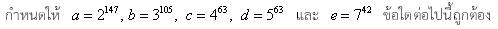# Comparison Mania

Algebra Level 3Let

$a = 2^{147}$

$b = 3^{105}$

$c = 4^{63}$

$d = 5^{63}$

$e = 7^{42}$

Arrange the values in descending order. Give your answer in the following form:

If you get $C > A > D > E > B$, your answer would be 31452.

If you get $A > E > B > D > C$, your answer would be 15243.

(I did not come up with this question. This is from the entrance exam of the Computer POSN camp in Thailand.)

×